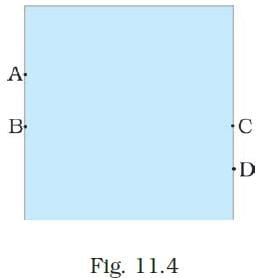UPSC  >  Test: Force & Pressure- 1

# Test: Force & Pressure- 1

Test Description

## 10 Questions MCQ Test Science & Technology for UPSC CSE | Test: Force & Pressure- 1

Test: Force & Pressure- 1 for UPSC 2023 is part of Science & Technology for UPSC CSE preparation. The Test: Force & Pressure- 1 questions and answers have been prepared according to the UPSC exam syllabus.The Test: Force & Pressure- 1 MCQs are made for UPSC 2023 Exam. Find important definitions, questions, notes, meanings, examples, exercises, MCQs and online tests for Test: Force & Pressure- 1 below.
Solutions of Test: Force & Pressure- 1 questions in English are available as part of our Science & Technology for UPSC CSE for UPSC & Test: Force & Pressure- 1 solutions in Hindi for Science & Technology for UPSC CSE course. Download more important topics, notes, lectures and mock test series for UPSC Exam by signing up for free. Attempt Test: Force & Pressure- 1 | 10 questions in 10 minutes | Mock test for UPSC preparation | Free important questions MCQ to study Science & Technology for UPSC CSE for UPSC Exam | Download free PDF with solutions
 1 Crore+ students have signed up on EduRev. Have you?
Test: Force & Pressure- 1 - Question 1

### A push or pull on an object is called

Detailed Solution for Test: Force & Pressure- 1 - Question 1

A push or pull on an object is called force. Force can make stationary body in motion and moving body in rest.

Test: Force & Pressure- 1 - Question 2

### Which one of the following forces is a contact force?

Detailed Solution for Test: Force & Pressure- 1 - Question 2

Contact forces are those that result when the two interacting objects are perceived to be physically contacting each other.
Example: Frictional forces, tensional forces, normal forces, air resistance forces, and applied forces.

Test: Force & Pressure- 1 - Question 3

### S.I. unit of force is

Detailed Solution for Test: Force & Pressure- 1 - Question 3

S.I. unit of force is Newton.
One Newton is a force required to push a body of mass of one kg to a distance of one meter.

Test: Force & Pressure- 1 - Question 4

In game of tug-of war, two teams

Detailed Solution for Test: Force & Pressure- 1 - Question 4

In a game of tug-of war, two teams pull the rope in opposite direction. The team that pulls the rope toward itself will become winner.

Test: Force & Pressure- 1 - Question 5

Perpendicular force acting per unit area is called

Detailed Solution for Test: Force & Pressure- 1 - Question 5

The total force acting perpendicular on a given surface is called thrust. Thrust acting upon a unit area is called pressure.

Test: Force & Pressure- 1 - Question 6

A water tank has four taps fixed at points A, B, C, D as shown in Fig. 11.4. The water will flow out at the same pressure from taps atDetailed Solution for Test: Force & Pressure- 1 - Question 6

In the given water tank, B and C are at the same levels. Hence, from taps B and C, water will flow out at the same pressure.

Test: Force & Pressure- 1 - Question 7

The net force on an object is __________ if the two forces acting on it in opposite directions are equal.

Detailed Solution for Test: Force & Pressure- 1 - Question 7

The force on an object is zero if the two forces acting on it in opposite directions are equal in magnitude. This kind of force is called balanced force.

Test: Force & Pressure- 1 - Question 8

A force of 16N acts on an area of 50 cm2. What is the pressure in pascal?

Detailed Solution for Test: Force & Pressure- 1 - Question 8

F = 16 N,
A = 50 cm2 = 50 / 10000 m2
We know that, P = F / A
∴ P = (16 x 10000) / 50 = 3200 Pa

Test: Force & Pressure- 1 - Question 9

A force applied on an object may change its

Detailed Solution for Test: Force & Pressure- 1 - Question 9

A force applied on an object may change its speed.

If the force applied on the object is in the direction of its motion, the speed of the object increases.
If the force applied on the object is in the direction opposite to the direction of motion, the speed of the object decreases.

Test: Force & Pressure- 1 - Question 10

A force of 1200 N acts on the surface of an area 10 cm2 normally. What would be the thrust and pressure on the surface?

Detailed Solution for Test: Force & Pressure- 1 - Question 10

Force, F = 1200 N
Area, A = 10 cm2 = 10×10-4 m2 = 10-3 m2

Thrust (perpendicular force), F = 1200 N

Pressure, P =  F/A = 1200/10−3 = 1.2×106 N/m2

## Science & Technology for UPSC CSE

85 videos|355 docs|218 tests
Information about Test: Force & Pressure- 1 Page
In this test you can find the Exam questions for Test: Force & Pressure- 1 solved & explained in the simplest way possible. Besides giving Questions and answers for Test: Force & Pressure- 1, EduRev gives you an ample number of Online tests for practice

## Science & Technology for UPSC CSE

85 videos|355 docs|218 tests

### How to Prepare for UPSC

Read our guide to prepare for UPSC which is created by Toppers & the best Teachers(Scan QR code)